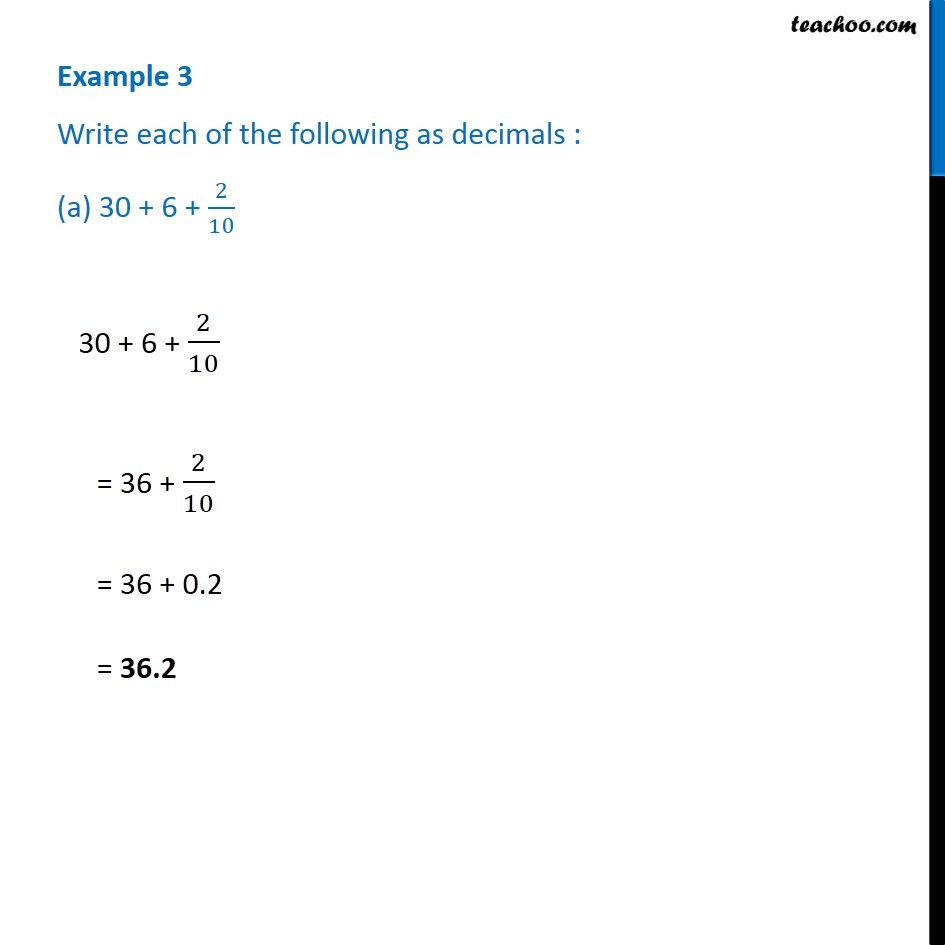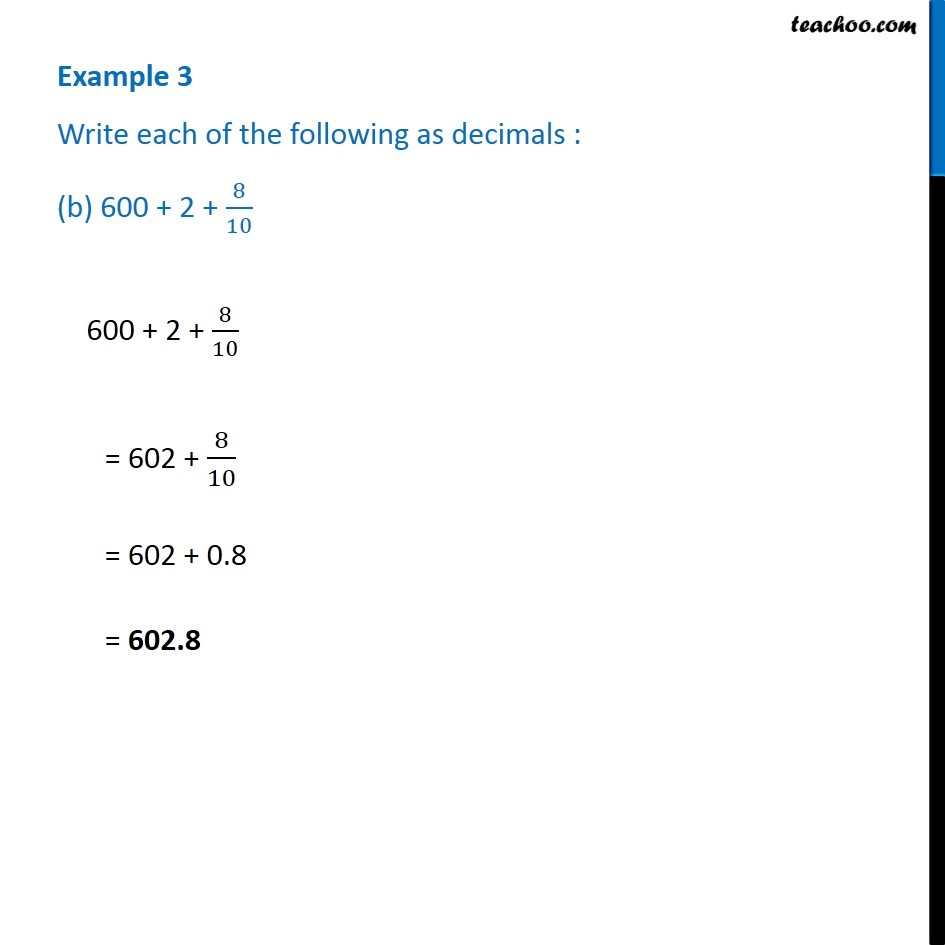Subscribe to our Youtube Channel - https://you.tube/teachoo

1. Chapter 8 Class 6 Decimals
2. Serial order wise
3. Examples

Transcript

Example 3 Write each of the following as decimals : (a) 30 + 6 + 2/10 30 + 6 + 2/10 = 36 + 2/10 = 36 + 0.2 = 36.2 Example 3 Write each of the following as decimals : (b) 600 + 2 + 8/10 600 + 2 + 8/10 = 602 + 8/10 = 602 + 0.8 = 602.8

Examples

Chapter 8 Class 6 Decimals
Serial order wise